# Rank charge density of spheres

• isukatphysics69
In summary, three conducting spheres are shown, with Sphere 3 initially having the highest net positive charge. After moving Sphere 2 towards Sphere 1, the magnitude of net charge ranks as 3>2=1. The charge density at labeled points is ranked as E>A>B=C=0>D, with positive charge ranked higher than negative charge.

## Homework Statement

Sphere 1 has net positive charge Sphere 2 has net negative charge Sphere 3 has net positive charge

The ranking of net charge magnitudes are
SPHERE 3 > SPHERE 2 > SPHERE 1

All spheres are conductors

Sphere 2 is moved away from Sphere 1 and toward Sphere 3 so that 2 and 3 touch.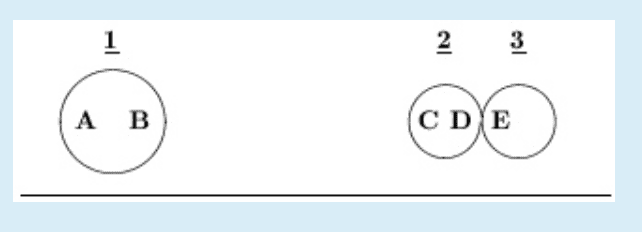Rank the charge density at each labeled point A -- E, greatest first. (Note that point E indicates the left side of Sphere 3; there is no labeled point on the right side of Sphere 3.)

Rank positive charge greater than negative charge. Include zero in your ranking.

HINT: Image what would happen is Sphere 1 were removed; then, consider what effect Sphere 1 has on Spheres 2 and 3 when it is present.

None

## The Attempt at a Solution

Sphere 3 has net positive charge and has the highest magnitude of net charge, sphere 3 is pushing the positives in sphere 2 to point C and since sphere 3 has the most net charge points E and D now have net positive charge, sphere 1 is net positive so it is pushing the positives in sphere 2 towards sphere 3 so I think C, D & E are all positively charged now and all the negative charged has transferred to the right corner of sphere 3. since sphere 1 is net positive I feel A and B are also positively charged

#### Attachments

isukatphysics69 said:

## Homework Statement

Sphere 1 has net positive charge Sphere 2 has net negative charge Sphere 3 has net positive charge

The ranking of net charge magnitudes are
SPHERE 3 > SPHERE 2 > SPHERE 1

All spheres are conductors

Sphere 2 is moved away from Sphere 1 and toward Sphere 3 so that 2 and 3 touch.
View attachment 230361

Rank the charge density at each labeled point A -- E, greatest first. (Note that point E indicates the left side of Sphere 3; there is no labeled point on the right side of Sphere 3.)

Rank positive charge greater than negative charge. Include zero in your ranking.

HINT: Image what would happen is Sphere 1 were removed; then, consider what effect Sphere 1 has on Spheres 2 and 3 when it is present.

None

## The Attempt at a Solution

Sphere 3 has net positive charge and has the highest magnitude of net charge, sphere 3 is pushing the positives in sphere 2 to point C and since sphere 3 has the most net charge points E and D now have net positive charge, sphere 1 is net positive so it is pushing the positives in sphere 2 towards sphere 3 so I think C, D & E are all positively charged now and all the negative charged has transferred to the right corner of sphere 3. since sphere 1 is net positive I feel A and B are also positively charged
What were the initial positions of the spheres?

SammyS said:
What were the initial positions of the spheres?
initially 2 was in the middle, 1 was far left 3 was far right,
then
2 was touching 1 and was moved away towards 3

Need halp

isukatphysics69 said:
initially 2 was in the middle, 1 was far left 3 was far right,
then
2 was touching 1 and was moved away towards 3
Seems like this is the tail end of a post which has been either removed from view or deleted.

If that's so, can you at least summarize the results of those parts?

•isukatphysics69
SammyS said:
Seems like this is the tail end of a post which has been either removed from view or deleted.

If that's so, can you at least summarize the results of those parts?
Yes, I will be back momentarily with the results

SammyS said:
Seems like this is the tail end of a post which has been either removed from view or deleted.

If that's so, can you at least summarize the results of those parts?
Three conducting spheres -- 1, 2, and 3 -- are shown below. Spheres 1 and 2 are initially neutral and Sphere 3 initially has a net positive charge. Spheres 2 and 3 are the same size, but Sphere 1 is larger, as indicated in the diagram. Note also that the center of Sphere 1 is about 10 times farther from the center of Sphere 3 as the radius of Sphere 3.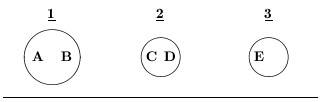Rank the charge density at each labeled point A -- E, greatest first. Input your answer in the format A>B=0>C>D=E.

Rank positive charge greater than negative charge. Include zero in your ranking.

Sphere 2 is moved toward Sphere 1 so that they touch.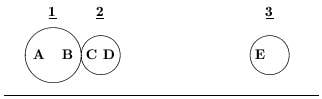Rank the magnitude of the net charge on each of the three spheres 1 -- 3 in the previous image, greatest first. Here, magnitude means absolute value, so ignore the sign of the charge.

Rank the charge density at each labeled point A -- E, greatest first. Input your answer in the format A>B=0>C>D=E.

Rank positive charge greater than negative charge. Include zero in your ranking.

Sphere 2 is moved away from Sphere 1 and toward Sphere 3 so that 2 and 3 touch.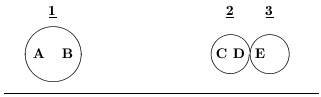Rank the magnitude of the net charge on each of the three spheres 1 -- 3, greatest first. Here, magnitude means absolute value, so ignore the sign of the charge.

Rank the charge density at each labeled point A -- E, greatest first. (Note that point E indicates the left side of Sphere 3; there is no labeled point on the right side of Sphere 3.)

Rank positive charge greater than negative charge. Include zero in your ranking.

HINT: Image what would happen is Sphere 1 were removed; then, consider what effect Sphere 1 has on Spheres 2 and 3 when it is present.

CORRECT ANSWER: THIS IS WHERE I AM COMPLETELY STUCK

#### Attachments

isukatphysics69 said:
Three conducting spheres -- 1, 2, and 3 -- are shown below. Spheres 1 and 2 are initially neutral and Sphere 3 initially has a net positive charge. Spheres 2 and 3 are the same size, but Sphere 1 is larger, as indicated in the diagram. Note also that the center of Sphere 1 is about 10 times farther from the center of Sphere 3 as the radius of Sphere 3.
...

Sphere 2 is moved away from Sphere 1 and toward Sphere 3 so that 2 and 3 touch.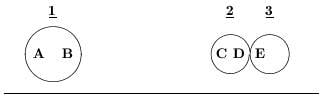Rank the magnitude of the net charge on each of the three spheres 1 -- 3, greatest first. Here, magnitude means absolute value, so ignore the sign of the charge.

Rank the charge density at each labeled point A -- E, greatest first. (Note that point E indicates the left side of Sphere 3; there is no labeled point on the right side of Sphere 3.)

Rank positive charge greater than negative charge. Include zero in your ranking.

HINT: Image what would happen is Sphere 1 were removed; then, consider what effect Sphere 1 has on Spheres 2 and 3 when it is present.

CORRECT ANSWER: THIS IS WHERE I AM COMPLETELY STUCK
I'm surprised that the answer given for magnitude in this case is correct. I would have Sphere2 > Sphere3 > Sphere1

Use the hint(s). The first part seems straight forward.

It seems clear to me which point has the greatest (positive) charge density What do you think it may be?

#### Attachments

•isukatphysics69
SammyS said:
I'm surprised that the answer given for magnitude in this case is correct. I would have Sphere2 > Sphere3 > Sphere1

Use the hint(s). The first part seems straight forward.

It seems clear to me which point has the greatest (positive) charge density What do you think it may be?
I think E has the greatest charge density

isukatphysics69 said:
I think E has the greatest charge density
Why is that?

Notice the answers for Spheres 1 and 2 touching?

•isukatphysics69
SammyS said:
Why is that?

Notice the answers for Spheres 1 and 2 touching?
I was thinking that E is the greatest because 3 has the greatest net charge and it is a positively charged sphere

My thinking was E>C>B>0>A>D

Sphere 3 has greatest net charge and positive, so it is E with the greatest, then that positive charge is pushing the positives in sphere 2 to the left so C is also positive but now as I am typing this I think B is before C

isukatphysics69 said:
I was thinking that E is the greatest because 3 has the greatest net charge and it is a positively charged sphere
Yes, but 2 and 5 are touhing and they're conductors. What effect does 1 have on them?

•isukatphysics69
SammyS said:
Yes, but 2 and 5 are touhing and they're conductors. What effect does 1 have on them?
1 is polorizing them pushing the positives to the right

So where do the negatives in the net negative sphere 2 go? its so confusing because you have 1 polorizing from the left and 3 polorizing on the right but 3 is more powerful so it should be pushing the positives to C but I don't know what happens to the negatives from 2, do they just go to the right corner of 3?

isukatphysics69 said:
1 is polarizing them pushing the positives to the right
Oh ! I mis-read the answer to the case of 1 and 2 touching.

•isukatphysics69
isukatphysics69 said:
My thinking was E>C>B>0>A>D

Sphere 3 has greatest net charge and positive, so it is E with the greatest, then that positive charge is pushing the positives in sphere 2 to the left so C is also positive but now as I am typing this I think B is before C
I doubt that A is negative, likewise doubt that B is positive. The side of Sphere 1 closest to the Spheres 2&3 should have positive (like) charge driven to the far side and negative (unlike) charge attracted.

•isukatphysics69
SammyS said:
I doubt that A is negative, likewise doubt that B is positive. The side of Sphere 1 closest to the Spheres 2&3 should have positive (like) charge driven to the far side and negative (unlike) charge attracted.
Im wondering what happens to the negatives from the net negatively charged sphere 2?

Im getting really pissed off at physics right now

isukatphysics69 said:
Im wondering what happens to the negatives from the net negatively charged sphere 2?
They are neutralized by some of the positive charges which make up the net positive charge of Sphere 3. After all, they're conductor making contact..

•isukatphysics69
Since 2 and 3 touch and have exact same geometry, I expect 2 and 3 to have exact same charges (both positive if understood correctly the previous processes that lead to this setup). But after we examine the effect of sphere 1, 2 will have abit less positive charge and 3 abit more positive charge since the net electric field inside 2 and 3 must cancel the electric field from sphere 1. So indeed it will be 3>2>1 for the charge.

And for the charge densities, it will be C>A>D=E=0>B

•isukatphysics69
SammyS said:
They are neutralized by some of the positive charges which make up the net positive charge of Sphere 3. After all, they're conductor making contact..
So my understanding at this time is all of points are positive. My professor told me earlier that the combination of spheres 2 and 3 are not polorizing sphere 1 enough to make point B negative, I was just confused about the contact between them because I don't know how much more sphere 3 is positive than sphere 2 is negative and I didn't know if it was only slightly more it would be able to neutralize

Delta² said:
Since 2 and 3 touch and have exact same geometry, I expect 2 and 3 to have exact same charges (both positive if understood correctly the previous processes that lead to this setup). But after we examine the effect of sphere 1, 2 will have abit less positive charge and 3 abit more positive charge since the net electric field inside 2 and 3 must cancel the electric field from sphere 1. So indeed it will be 3>2>1 for the charge.

And for the charge densities, it will be C>A>D=E=0>B
Hey man thank you for your time but please do not tell the answers, that is incorrect but in the future please do not just tell the answer thank you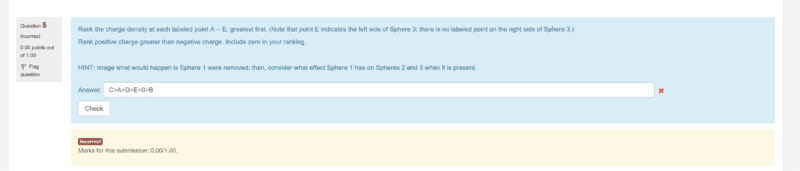#### Attachments

•Delta2
Ok I am sorry, can you tell me the web page to check myself, I did the mistake of taking B as negative but now I realized this is not the case. (and also D=E=0 doesn't look quite correct ).

•isukatphysics69
Delta² said:
Ok I am sorry, can you tell me the web page to check myself, I did the mistake of taking B as negative but now I realized this is not the case. (and also D=E=0 doesn't look quite correct ).
I am logged into my personal moodle account so I cannot share the web page unfortunately

right now I was thinking E>D>C>A>B>0 but that was also incorrect

I was thinking C>A>B>E=D=0 but hard to explain my reasoning.

I ask for this : What is the net charge of sphere 2 on her way to touch the sphere 3 and before the touch. If I understood correctly sphere 2 has negative charge and in absolute value smaller than the positive charge of sphere 3. Is that correct?

•isukatphysics69
Look at part 1 of the hint again.

•isukatphysics69
Delta² said:
I was thinking C>A>B>E=D=0 but hard to explain my reasoning.

I ask for this : What is the net charge of sphere 2 on her way to touch the sphere 3 and before the touch. If I understood correctly sphere 2 has negative charge and in absolute value smaller than the positive charge of sphere 3. Is that correct?
Incorrect

@SammyS I think I know what you are saying, so I am only looking at half of the charge in sphere 3 so E = C = D?

Nvm that is wrong too

how does C > D = E sound

Delta² said:
I was thinking C>A>B>E=D=0 but hard to explain my reasoning.

I ask for this : What is the net charge of sphere 2 on her way to touch the sphere 3 and before the touch. If I understood correctly sphere 2 has negative charge and in absolute value smaller than the positive charge of sphere 3. Is that correct?
Also yes you are correct with reasoning but not answer

•Delta2
isukatphysics69 said:
Also yes you are correct with reasoning but not answer

You mean I am correct as to my comment on the net charge of sphere 2 before it touches sphere 3?

Anyway I haven't run into many problems of this type so I ll stop of making guesses . I think this problem is made to check the understanding of the electrostatic induction and the equipotential process when the spheres touch.

•isukatphysics69
Delta² said:
You mean I am correct as to my comment on the net charge of sphere 2 before it touches sphere 3?

Anyway I haven't run into many problems of this type so I ll stop of making guesses . I think this problem is made to check the understanding of the electrostatic induction and the equipotential process when the spheres touch.
yes that's what I meant an yea that's what this problem is I will drop out and work on cargo ship this is too complicated

•Delta2
isukatphysics69 said:
Incorrect

@SammyS I think I know what you are saying, so I am only looking at half of the charge in sphere 3 so E = C = D?

Nvm that is wrong too

how does C > D = E sound

•isukatphysics69
C > D = E makes sense if we remove Sphere 1. The majority of excess charge mill migrate to the far ends the 2,3 combination, that is C and the unmarked right side of 3. Density at D,E will be much less.

Then, bring Sphere 1 back. Remember, its still relatively far away and has the smallest net charge.

I suspect the right hand side of 3 has largest density, then C > A ?? E > D ?? >0 not sure where B goes.

Maybe between A & E, else between D and 0 .

•isukatphysics69 and Delta2
SammyS said:
C > D = E makes sense if we remove Sphere 1. The majority of excess charge mill migrate to the far ends the 2,3 combination, that is C and the unmarked right side of 3. Density at D,E will be much less.

Then, bring Sphere 1 back. Remember, its still relatively far away and has the smallest net charge.

I suspect the right hand side of 3 has largest density, then C > A ?? E > D ?? >0 not sure where B goes.

Maybe between A & E, else between D and 0 .
Thank you for reply, I will read tomorrow and give this another shot. 8 hours spent on this today and got nowhere I think I am done for today